# Rectangle

Rectangle
Rectangle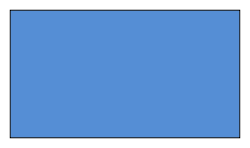Family Orthotope
Type Quadrilateral
Edges and vertices 4
Schläfli symbol {}x{}
Symmetry group D2, , (*22)
Coxeter-Dynkin diagramDual polygon Rhombus
Properties isogonal, convex, cyclic

In Euclidean plane geometry, a rectangle is any quadrilateral with four right angles. The term "oblong" is occasionally used to refer to a non-square rectangle. A rectangle with vertices ABCD would be denoted as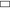ABCD.

The word rectangle comes from the Latin "rectangulus", which is a combination of "rectus" (right) and "angulus" (angle).

A so-called crossed rectangle is a crossed (self-intersecting) quadrilateral which consists of two opposite sides of a rectangle along with the two diagonals. It is a special case of an antiparallelogram, and its angles are not right angles. Other geometries, such as spherical, elliptic, and hyperbolic, have so-called rectangles with opposite sides equal in length and equal angles that are not right angles.

Rectangles are involved in many tiling problems, such as tiling the plane by rectangles or tiling a rectangle by polygons.

## Characterizations

A convex quadrilateral is a rectangle if and only if it is any one of the following:

• a parallelogram with at least one right angle
• an equiangular parallelogram
• a parallelogram with congruent diagonals
• a parallelogram ABCD where triangles ABD and DCA are congruent
• a quadrilateral which has four right angles
• an equiangular quadrilateral

## Classification

### Traditional hierarchy

A rectangle is a special case of a parallelogram in which each pair of adjacent sides is perpendicular.

A parallelogram, and hence also a rectangle, is a special case of a trapezium (known as a trapezoid in North America) in which both pairs of opposite sides are parallel and equal in length.

A trapezium, and hence also a rectangle, is a convex quadrilateral which has at least one pair of parallel opposite sides.

A convex quadrilateral, and hence also a rectangle, is

• Star-shaped: The whole interior is visible from a single point, without crossing any edge.
• Simple: The boundary does not cross itself.

### Alternative hierarchy

De Villiers defines a rectangle more generally as any quadrilateral with axes of symmetry through each pair of opposite sides. This definition includes both right-angled rectangles and crossed rectangles. Each has an axis of symmetry parallel to and equidistant from a pair of opposite sides, and another which is the perpendicular bisector of those sides, but, in the case of the crossed rectangle, the first axis is not an axis of symmetry for either side that it bisects.

Quadrilaterals with two axes of symmetry, each through a pair of opposite sides, belong to the larger class of quadrilaterals with at least one axis of symmetry through a pair of opposite sides. These quadrilaterals comprise isosceles trapezia and crossed isosceles trapezia (crossed quadrilaterals with the same vertex arrangement as isosceles trapezia).

## Properties

### Symmetry

A rectangle is cyclic: all corners lie on a single circle.

It is equiangular: all its corner angles are equal (each of 90 degrees).

It is isogonal or vertex-transitive: all corners lie within the same symmetry orbit.

It has two lines of reflectional symmetry and rotational symmetry of order 2 (through 180°).

### Rectangle-rhombus duality

The dual polygon of a rectangle is a rhombus, as shown in the table below.

Rectangle Rhombus
All angles are congruent. All sides are congruent.
Its centre is equidistant from its vertices, hence it has a circumcircle. Its centre is equidistant from its sides, hence it has an incircle.
Its axes of symmetry bisect opposite sides. Its axes of symmetry bisect opposite angles.

### Miscellaneous

The two diagonals are equal in length and bisect each other. Every quadrilateral with both these properties is a rectangle.

A rectangle is rectilinear: its sides meet at right angles.

A non-square rectangle has 5 degrees of freedom, comprising 2 for position, 1 for rotational orientation, 1 for overall size, and 1 for shape.

Two rectangles, neither of which will fit inside the other, are said to be incomparable.

With vertices denoted A, B, C, and D, for any point P in the interior of a rectangle, (AP)2 + (CP)2 = (BP)2 + (DP)2.

## Formulas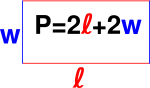The formula for the perimeter of a rectangle.

If a rectangle has length l and width w

• it has area A = lw,
• it has perimeter P = 2l + 2w = 2(l + w),
• each diagonal has length$\sqrt{l^2 + w^2}$,
• and when l = w, the rectangle is a square.

## Theorems

The isoperimetric theorem for rectangles states that among all rectangles of a given perimeter, the square has the largest area.

The midpoints of the sides of any quadrilateral with perpendicular diagonals form a rectangle.

A parallelogram with equal diagonals is a rectangle.

The Japanese theorem for cyclic quadrilaterals states that the incentres of the four triangles determined by the vertices of a cyclic quadrilateral taken three at a time form a rectangle.

## Crossed rectangles

A crossed (self-intersecting) quadrilateral consists of two opposite sides of a non-self-intersecting quadrilateral along with the two diagonals. Similarly, a crossed rectangle is a crossed quadrilateral which consists of two opposite sides of a rectangle along with the two diagonals. It has the same vertex arrangement as the rectangle. It appears as two identical triangles with a common vertex, but the geometric intersection is not considered a vertex.

A crossed quadrilateral is sometimes likened to a bow tie or butterfly. A three-dimensional rectangular wire frame that is twisted can take the shape of a bow tie. A crossed rectangle is sometimes called an "angular eight".

The interior of a crossed rectangle can have a polygon density of +/-1 in each triangle, dependent upon the winding orientation as clockwise or counterclockwise.

A crossed rectangle is not equiangular. The sum of its interior angles (two acute and two reflex), as with any crossed quadrilateral, is 720°.

A rectangle and a crossed rectangle are quadrilaterals with the following properties in common:

• Opposite sides are equal in length.
• The two diagonals are equal in length.
• It has two lines of reflectional symmetry and rotational symmetry of order 2 (through 180°).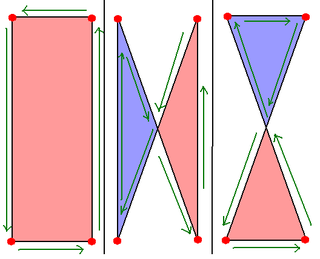## Other rectangles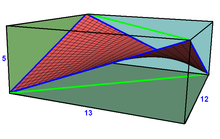A saddle rectangle has 4 nonplanar vertices, alternated from vertices of a cuboid, with a unique minimal surface interior defined as a linear combination of the four vertices, creating a saddle surface. This example shows 4 blue edges of the rectangle, and two green diagonals, all being diagonal of the cuboid rectangular faces.

In solid geometry, a figure is non-planar if it is not contained in a (flat) plane. A skew rectangle is a non-planar quadrilateral with opposite sides equal in length and four equal acute angles.[citation needed] A saddle rectangle is a skew rectangle with vertices that alternate an equal distance above and below a plane passing through its center, named for its minimal surface interior seen with saddle point at its center. The convex hull of this skew rectangle is a special tetrahedron called a rhombic disphenoid. (The term "skew rectangle" is also used in 2D graphics to refer to a distortion of a rectangle using a "skew" tool. The result can be a parallelogram or a trapezoid/trapezium.)

In spherical geometry, a spherical rectangle is a figure whose four edges are great circle arcs which meet at equal angles greater than 90 degrees. Opposite arcs are equal in length. The surface of a sphere in Euclidean solid geometry is a non-Euclidean surface in the sense of elliptic geometry. Spherical geometry is the simplest form of elliptic geometry.

In elliptic geometry, an elliptic rectangle is a figure in the elliptic plane whose four edges are elliptic arcs which meet at equal angles greater than 90 degrees. Opposite arcs are equal in length.

In hyperbolic geometry, a hyperbolic rectangle is a figure in the hyperbolic plane whose four edges are hyperbolic arcs which meet at equal angles less than 90 degrees. Opposite arcs are equal in length.

## Tessellations

The rectangle is used in many periodic tessellation patterns, in brickwork, for example, these tilings:

## Squared, perfect, and other tiled rectangles

A rectangle tiled by squares, rectangles, or triangles is said to be a "squared", "rectangled", or "triangulated" (or "triangled") rectangle respectively. The tiled rectangle is perfect if the tiles are similar and finite in number and no two tiles are the same size. If two such tiles are the same size, the tiling is imperfect. In a perfect (or imperfect) triangled rectangle the triangles must be right triangles.

A rectangle has commensurable sides if and only if it is tileable by a finite number of unequal squares. The same is true if the tiles are unequal isosceles right triangles.

The tilings of rectangles by other tiles which have attracted the most attention are those by congruent non-rectangular polyominoes, allowing all rotations and reflections. There are also tilings by congruent polyaboloes.

## See also

Wikimedia Foundation. 2010.

### Look at other dictionaries:

• rectangle — [ rɛktɑ̃gl ] adj. et n. m. • 1549; lat. rectangulus, de rectus « droit » et angulus « angle » I ♦ Adj. Didact. 1 ♦ Géom. Dont un angle au moins est droit. Triangle, trapèze rectangle. Parallélépipède rectangle : prisme droit dont les bases sont… …   Encyclopédie Universelle

• Rectangle — Rec tan gle (r?k t?? g l), n. [F., fr. L. rectus right + angulus angle. See {Right}, and {Angle}.] (Geom.) A four sided figure having only right angles; a right angled parallelogram. [1913 Webster] Note: As the area of a rectangle is expressed by …   The Collaborative International Dictionary of English

• rectangle — Rectangle. adj. de tout genre. Qui a un ou plusieurs angles droits. Triangle rectangle. parallelograme rectangle …   Dictionnaire de l'Académie française

• rectangle — 1570s, from M.Fr. rectangle, from rect , comb. form of L. rectus right (see RIGHT (Cf. right) (adj.1)) + O.Fr. angle (see ANGLE (Cf. angle)). M.L. rectangulum meant a triangle having a right angle (7c.) …   Etymology dictionary

• Rectangle — Rec tan gle, a. Rectangular. [R.] [1913 Webster] …   The Collaborative International Dictionary of English

• rectangle — ► NOUN ▪ a plane figure with four straight sides and four right angles, and with unequal adjacent sides. DERIVATIVES rectangular adjective. ORIGIN Latin rectangulum, from rectus straight + angulus an angle …   English terms dictionary

• rectangle — [rek′taŋ΄gəl] n. [Fr < ML rectangulum < L rectus (see RECTI ) + angulus,ANGLE1] 1. any four sided plane figure with four right angles: see QUADRILATERAL 2. any such figure or shape that is not a square; oblong …   English World dictionary

• Rectangle — Un rectangle. En géométrie, un rectangle est un quadrilatère dont les quatre angles sont des angles droits. Sommaire 1 Déf …   Wikipédia en Français

• rectangle — (rè ktan gl ) adj. Terme de géométrie. Qui a les angles droits. Une figure rectangle.    S. m. Un rectangle, un parallélogramme rectangle. Tracer un rectangle.    Produit de deux lignes quelconques de grandeurs différentes. ÉTYMOLOGIE    Lat.… …   Dictionnaire de la Langue Française d'Émile Littré

• RECTANGLE — adj. des deux genres T. de Géom. Il se dit, soit D un triangle qui a un angle droit, soit D un parallélogramme qui a quatre angles droits. Un triangle rectangle. Un parallélogramme rectangle.   Il s emploie aussi substantivement, et signifie, Un… …   Dictionnaire de l'Academie Francaise, 7eme edition (1835)

### Share the article and excerpts

##### Direct link
Do a right-click on the link above
and select “Copy Link”